# 评估自动化机器学习试验结果Evaluate automated machine learning experiment results

## 查看运行结果View run results

1. 登录到工作室并导航到你的工作区。Sign into the studio and navigate to your workspace.
2. 在左侧菜单中选择“试验”。In the left menu, select Experiments.
3. 从试验列表中选择你的试验。Select your experiment from the list of experiments.
4. 在页面底部的表中，选择自动化 ML 运行。In the table at the bottom of the page, select an automated ML run.
5. 在“模型”选项卡中，选择要评估的模型的“算法名称” 。In the Models tab, select the Algorithm name for the model you want to evaluate.
6. 在“指标”选项卡中，使用左侧的复选框查看指标和图表。In the Metrics tab, use the checkboxes on the left to view metrics and charts.## 分类指标Classification metrics

• - 计算每个类的指标并取未加权平均值Macro - Calculate the metric for each class and take the unweighted average
• Micro - 通过统计真正、假负和假正总值（独立于类）来全局计算指标。Micro - Calculate the metric globally by counting the total true positives, false negatives, and false positives (independent of classes).
• 加权 - 计算每个类的指标，并根据每个类的样本数取加权平均值。Weighted - Calculate the metric for each class and take the weighted average based on the number of samples per class.

AUCAUC AUC 是接收方操作特性曲线下面的区域。AUC is the Area under the Receiver Operating Characteristic Curve.

• `AUC_macro`，每个类的 AUC 算术平均值。`AUC_macro`, the arithmetic mean of the AUC for each class.
• `AUC_micro`，通过组合每个类中的真正和假正来计算 Micro。`AUC_micro`, computed by combining the true positives and false positives from each class.
• `AUC_weighted`，每个类的评分算术平均值，按每个类中的真实实例数加权。`AUC_weighted`, arithmetic mean of the score for each class, weighted by the number of true instances in each class.
• 计算Calculation
accuracyaccuracy Accuracy 是与真实类标签完全匹配的预测比率。Accuracy is the ratio of predictions that exactly match the true class labels.

目的： 越接近 1 越好Objective: Closer to 1 the better
范围： [0, 1]Range: [0, 1]
计算Calculation
average_precisionaverage_precision 平均精度以每个阈值实现的加权精度汇总精度-召回率曲线，使用前一阈值中的召回率增量作为权重。Average precision summarizes a precision-recall curve as the weighted mean of precisions achieved at each threshold, with the increase in recall from the previous threshold used as the weight.

目的： 越接近 1 越好Objective: Closer to 1 the better
范围： [0, 1]Range: [0, 1]

支持的指标名称包括，Supported metric names include,
• `average_precision_score_macro`，每个类的平均精度评分算术平均值。`average_precision_score_macro`, the arithmetic mean of the average precision score of each class.
• `average_precision_score_micro`，通过组合每个交接中的真正和假正来计算。`average_precision_score_micro`, computed by combining the true positives and false positives at each cutoff.
• `average_precision_score_weighted`，每个类的平均精度评分算术平均值，按每个类中的真实实例数加权。`average_precision_score_weighted`, the arithmetic mean of the average precision score for each class, weighted by the number of true instances in each class.
• 计算Calculation
balanced_accuracybalanced_accuracy 平衡准确度是每个类的召回率算术平均值。Balanced accuracy is the arithmetic mean of recall for each class.

目的： 越接近 1 越好Objective: Closer to 1 the better
范围： [0, 1]Range: [0, 1]
计算Calculation
f1_scoref1_score F1 评分是精度和召回率的调和平均值。F1 score is the harmonic mean of precision and recall. 这是一个很好的衡量假正和假负的平衡。It is a good balanced measure of both false positives and false negatives. 然而，它没有考虑到真负。However, it does not take true negatives into account.

目的： 越接近 1 越好Objective: Closer to 1 the better
范围： [0, 1]Range: [0, 1]

支持的指标名称包括，Supported metric names include,
• `f1_score_macro`：每个类的 F1 评分算术平均值。`f1_score_macro`: the arithmetic mean of F1 score for each class.
• `f1_score_micro`：通过统计真正、假负和假正总值来计算得出。`f1_score_micro`: computed by counting the total true positives, false negatives, and false positives.
• `f1_score_weighted`：按每个类的 F1 评分类频率计算的加权平均值。`f1_score_weighted`: weighted mean by class frequency of F1 score for each class.
• 计算Calculation
log_losslog_loss 这是（多项式） 逻辑回归及其扩展（例如神经网络）中使用的损失函数，在给定概率分类器的预测的情况下，定义为真实标签的负对数可能性。This is the loss function used in (multinomial) logistic regression and extensions of it such as neural networks, defined as the negative log-likelihood of the true labels given a probabilistic classifier's predictions.

目的： 越接近 0 越好Objective: Closer to 0 the better
范围： [0, inf)Range: [0, inf)
计算Calculation
norm_macro_recallnorm_macro_recall 规范化宏召回率是对宏召回率进行规范化和平均化，因此，随机性能的评分为 0，完美性能的评分为 1。Normalized macro recall is recall macro-averaged and normalized, so that random performance has a score of 0, and perfect performance has a score of 1.

目的： 越接近 1 越好Objective: Closer to 1 the better
范围： [0, 1]Range: [0, 1]
`(recall_score_macro - R)` / `(1 - R)`

其中，`R` 是随机预测的 `recall_score_macro` 期望值。where, `R` is the expected value of `recall_score_macro` for random predictions.

`R = 0.5`  表示 二进制 分类。`R = 0.5` for  binary classification.
`R = (1 / C)` 表示 C 类分类问题。`R = (1 / C)` for C-class classification problems.
matthews_correlationmatthews_correlation Matthews 关联系数是一种平衡准确性度量值，即使一个类比另一个类有更多的样本，也可以使用它。Matthews correlation coefficient is a balanced measure of accuracy, which can be used even if one class has many more samples than another. 系数 1 表示完美预测、0 表示随机预测以及 -1 表示反向预测。A coefficient of 1 indicates perfect prediction, 0 random prediction, and -1 inverse prediction.

目的： 越接近 1 越好Objective: Closer to 1 the better
范围： [-1, 1]Range: [-1, 1]
计算Calculation
精准率precision 精准率是模型避免将负样本标记为正样本的能力。Precision is the ability of a model to avoid labeling negative samples as positive.

目的： 越接近 1 越好Objective: Closer to 1 the better
范围： [0, 1]Range: [0, 1]

支持的指标名称包括，Supported metric names include,
• `precision_score_macro`，每个类的精度算术平均值。`precision_score_macro`, the arithmetic mean of precision for each class.
• `precision_score_micro`，通过统计真正和假正总值来全局计算 Micro。`precision_score_micro`, computed globally by counting the total true positives and false positives.
• `precision_score_weighted`，每个类的精度算术平均值，按每个类中的真实实例数加权。`precision_score_weighted`, the arithmetic mean of precision for each class, weighted by number of true instances in each class.
• 计算Calculation
召回率recall 召回率是模型检测所有正的样本。Recall is the ability of a model to detect all positive samples.

目的： 越接近 1 越好Objective: Closer to 1 the better
范围： [0, 1]Range: [0, 1]

支持的指标名称包括，Supported metric names include,
• `recall_score_macro`，每个类的召回率算术平均值。`recall_score_macro`: the arithmetic mean of recall for each class.
• `recall_score_micro`：通过统计真正、假负和假正总值来全局计算。`recall_score_micro`: computed globally by counting the total true positives, false negatives and false positives.
• `recall_score_weighted`：每个类的召回率算术平均值，按每个类中的真实实例数加权。`recall_score_weighted`: the arithmetic mean of recall for each class, weighted by number of true instances in each class.
• 计算Calculation
weighted_accuracyweighted_accuracy 加权准确性是指每个样本按属于同一类别的样本总数加权的准确性。Weighted accuracy is accuracy where each sample is weighted by the total number of samples belonging to the same class.

目的： 越接近 1 越好Objective: Closer to 1 the better
范围： [0, 1]Range: [0, 1]
计算Calculation

### 二进制与多类分类指标Binary vs. multiclass classification metrics

自动化 ML 不区分二元分类指标与多类指标。Automated ML doesn't differentiate between binary and multiclass metrics. 不管数据集有两个类还是两个以上的类，都会报告相同的验证指标。The same validation metrics are reported whether a dataset has two classes or more than two classes. 但是，某些指标旨在用于多类分类。However, some metrics are intended for multiclass classification. 正如你所期望的那样，这些指标在应用于二元分类数据集时不会将任何类视为 `true` 类。When applied to a binary dataset, these metrics won't treat any class as the `true` class, as you might expect. 明确用于多类的指标以 `micro``macro``weighted` 为后缀。Metrics that are clearly meant for multiclass are suffixed with `micro`, `macro`, or `weighted`. 示例包括 `average_precision_score``f1_score``precision_score``recall_score``AUC`Examples include `average_precision_score`, `f1_score`, `precision_score`, `recall_score`, and `AUC`.

例如，多类平均召回率（`micro``macro``weighted`）不按 `tp / (tp + fn)` 计算召回率，而是对二进制分类数据集的两个类进行平均。For example, instead of calculating recall as `tp / (tp + fn)`, the multiclass averaged recall (`micro`, `macro`, or `weighted`) averages over both classes of a binary classification dataset. 这相当于分别计算 `true` 类和 `false` 类的召回率，然后取二者的平均值。This is equivalent to calculating the recall for the `true` class and the `false` class separately, and then taking the average of the two.

自动化 ML 不会计算二进制指标（即二进制分类数据集的指标）。Automated ML doesn't calculate binary metrics, that is metrics for binary classification datasets. 但是，可以使用自动 ML 为该特定运行生成的混淆矩阵手动计算这些指标。However, these metrics can be manually calculated using the confusion matrix that Automated ML generated for that particular run. 例如，可以使用 2x2 混淆矩阵图中显示的真正值和假正值来计算精度，即 `tp / (tp + fp)`For example, you can calculate precision, `tp / (tp + fp)`, with the true positive and false positive values shown in a 2x2 confusion matrix chart.

## 混淆矩阵Confusion matrix

混淆矩阵提供了机器学习模型如何在分类模型的预测中产生系统性误差的直观信息。Confusion matrices provide a visual for how a machine learning model is making systematic errors in its predictions for classification models. 名称中的“混淆”一词来自模型“混淆”或错误标记样本。The word "confusion" in the name comes from a model "confusing" or mislabeling samples. 混淆矩阵中第`i` 行和第 `j` 列的单元格包含评估数据集中属于类 `C_i` 且由模型分类为类 `C_j` 的样本数。A cell at row `i` and column `j` in a confusion matrix contains the number of samples in the evaluation dataset that belong to class `C_i` and were classified by the model as class `C_j`.

在工作室中，较暗的单元格表示样本数较多。In the studio, a darker cell indicates a higher number of samples. 在下拉列表中选择规范化的视图将对每个矩阵行进行规范化，以显示预测为类 `C_j` 的类 `C_i` 的百分比。Selecting Normalized view in the dropdown will normalize over each matrix row to show the percent of class `C_i` predicted to be class `C_j`. 默认 Raw 视图的好处是，可以看到实际类分布的不平衡是否导致模型从少数类中错分类样本，这是不平衡数据集的常见问题。The benefit of the default Raw view is that you can see whether imbalance in the distribution of actual classes caused the model to misclassify samples from the minority class, a common issue in imbalanced datasets.

良好模型的混淆矩阵沿对角线方向的样本最多。The confusion matrix of a good model will have most samples along the diagonal.

### 良好模型的混淆矩阵Confusion matrix for a good model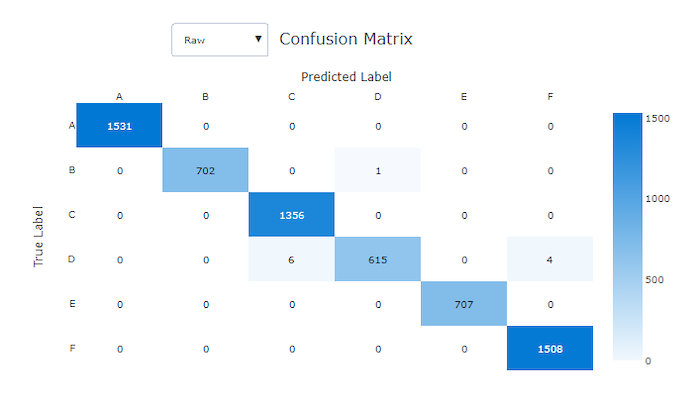### 不良模型的混淆矩阵Confusion matrix for a bad model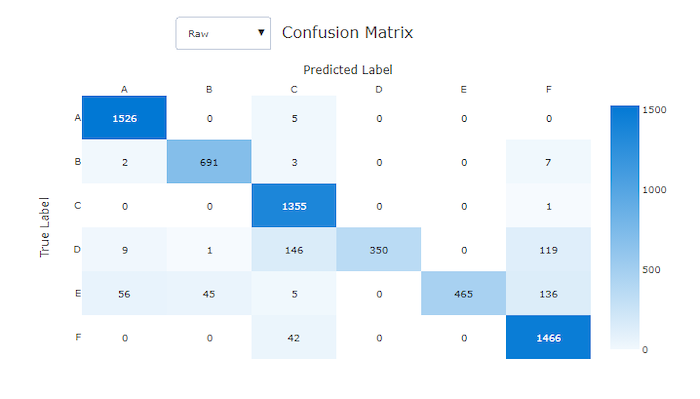## ROC 曲线ROC curve

接收方操作特征 (ROC) 曲线描绘了真正率 (TPR) 和假正率 (FPR) 随决策阈值变化的关系。The receiver operating characteristic (ROC) curve plots the relationship between true positive rate (TPR) and false positive rate (FPR) as the decision threshold changes. 在类失衡严重的情况下基于数据集训练模型时，ROC 曲线提供的信息可能较少，因为多数类可能会掩盖少数类的贡献。The ROC curve can be less informative when training models on datasets with high class imbalance, as the majority class can drown out contributions from minority classes.

曲线下区域 (AUC) 可以解释为正确分类样本的比例。The area under the curve (AUC) can be interpreted as the proportion of correctly classified samples. 更准确地说，AUC 是分类器对随机选择的正样本的排名高于随机选择的负样本的概率。More precisely, the AUC is the probability that the classifier ranks a randomly chosen positive sample higher than a randomly chosen negative sample. 曲线的形状直观地表明 TPR 和 FPR 之间的关系是分类阈值或决策边界的函数。The shape of the curve gives an intuition for relationship between TPR and FPR as a function of the classification threshold or decision boundary.

接近图表左上角的曲线接近 100% TPR 和 0% FPR，这是最佳模型。A curve that approaches the top-left corner of the chart is approaching a 100% TPR and 0% FPR, the best possible model. 随机模型会沿着 `y = x` 线从左下角到右上角生成一条 ROC 曲线。A random model would produce an ROC curve along the `y = x` line from the bottom-left corner to the top-right. 比随机模型更糟糕的模型，其 ROC 曲线会下降到 `y = x` 线以下。A worse than random model would have an ROC curve that dips below the `y = x` line.

提示

对于分类实验，为自动化 ML 模型生成的每个折线图都可以用于评估每个类的模型或所有类的平均值。For classification experiments, each of the line charts produced for automated ML models can be used to evaluate the model per-class or averaged over all classes. 通过单击图表右侧图例中的类标签，可以在这些不同的视图之间切换。You can switch between these different views by clicking on class labels in the legend to the right of the chart.

### 良好模型的 ROC 曲线ROC curve for a good model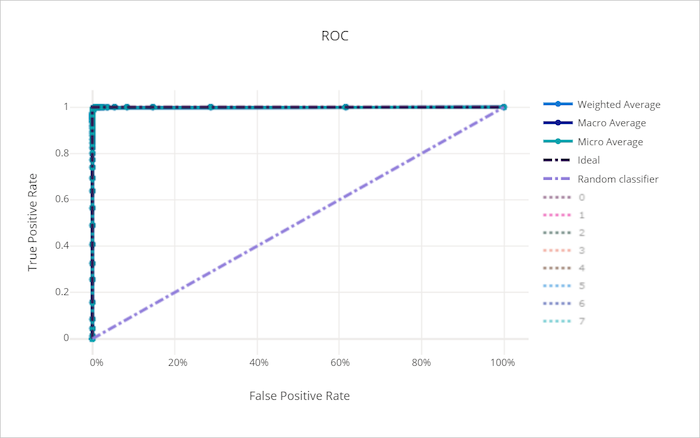### 不良模型的 ROC 曲线ROC curve for a bad model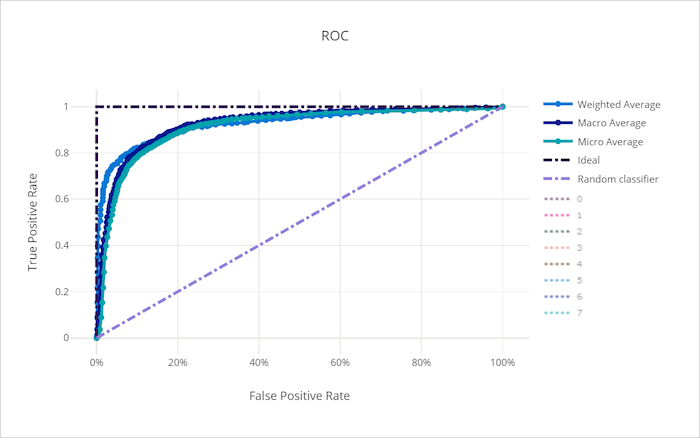## 精度-召回率曲线Precision-recall curve

精准率-召回率曲线描绘了精准率与召回率之间随决策阈值变化的关系。The precision-recall curve plots the relationship between precision and recall as the decision threshold changes. 召回率是模型检测所有正样本的能力，精准率是模型避免将负样本标记为正样本的能力。Recall is the ability of a model to detect all positive samples and precision is the ability of a model to avoid labeling negative samples as positive. 某些业务问题可能需要更高的召回率和更高的精准率，这取决于避免假负和假正的相对重要性。Some business problems might require higher recall and some higher precision depending on the relative importance of avoiding false negatives vs false positives.

提示

对于分类实验，为自动化 ML 模型生成的每个折线图都可以用于评估每个类的模型或所有类的平均值。For classification experiments, each of the line charts produced for automated ML models can be used to evaluate the model per-class or averaged over all classes. 通过单击图表右侧图例中的类标签，可以在这些不同的视图之间切换。You can switch between these different views by clicking on class labels in the legend to the right of the chart.

### 良好模型的精准率-召回率曲线Precision-recall curve for a good model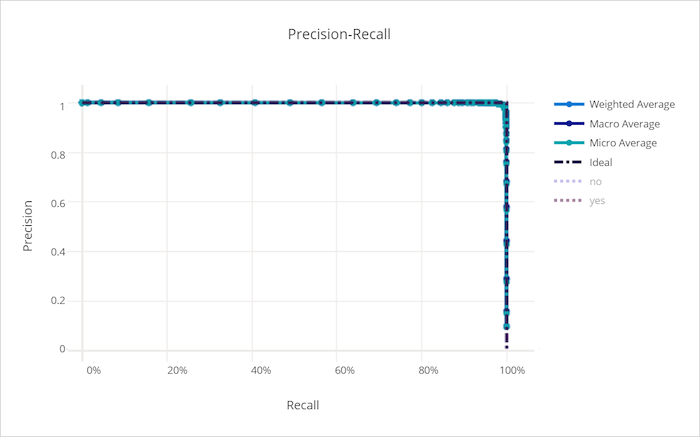### 不良模型的精准率-召回率曲线Precision-recall curve for a bad model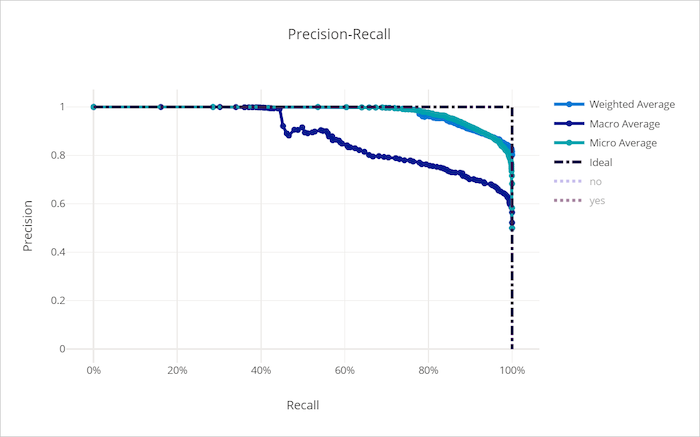## 累积增益曲线Cumulative gains curve

累积增益曲线绘制了正确分类的正样本百分比，作为我们按预测概率顺序考虑样本所占百分比的函数。The cumulative gains curve plots the percent of positive samples correctly classified as a function of the percent of samples considered where we consider samples in the order of predicted probability.

若要计算增益，首先从模型预测的最高概率到最低概率对所有样本进行排序。To calculate gain, first sort all samples from highest to lowest probability predicted by the model. 然后取 `x%` 的最高置信度预测值。Then take `x%` of the highest confidence predictions. 将在该 `x%` 中检测到的正样本数除以正样本总数得到增益。Divide the number of positive samples detected in that `x%` by the total number of positive samples to get the gain. 累积增益是我们在考虑最有可能属于正类别的某些数据百分比时检测到的正样本百分比。Cumulative gain is the percent of positive samples we detect when considering some percent of the data that is most likely to belong to the positive class.

理想的模型将所有正样本的排名高于所有负样本，给出由两个直线段组成的累积增益曲线。A perfect model will rank all positive samples above all negative samples giving a cumulative gains curve made up of two straight segments. 第一条是斜率为 `1 / x` 的线，从 `(0, 0)``(x, 1)`，其中 `x` 是属于正类的样本分数（如果类是平衡的，则为 `1 / num_classes`）。The first is a line with slope `1 / x` from `(0, 0)` to `(x, 1)` where `x` is the fraction of samples that belong to the positive class (`1 / num_classes` if classes are balanced). 第二条是从 `(x, 1)``(1, 1)` 的水平线。The second is a horizontal line from `(x, 1)` to `(1, 1)`. 在第一段中，所有正样本都已正确分类，累积增益在考虑的样本的第一个 `x%` 内到达 `100%`In the first segment, all positive samples are classified correctly and cumulative gain goes to `100%` within the first `x%` of samples considered.

基线随机模型在 `y = x` 之后有一个累积增益曲线，其中在所考虑的 `x%` 的样本中仅检测到 `x%` 的总正样本。The baseline random model will have a cumulative gains curve following `y = x` where for `x%` of samples considered only about `x%` of the total positive samples were detected. 完美模型会有一条触及左上角的微平均曲线和一条斜率 `1 / num_classes` 的宏平均线，直到累积增益达到 100%，然后水平延伸，直到数据百分比达到 100。A perfect model will have a micro average curve that touches the top-left corner and a macro average line that has slope `1 / num_classes` until cumulative gain is 100% and then horizontal until the data percent is 100.

提示

对于分类实验，为自动化 ML 模型生成的每个折线图都可以用于评估每个类的模型或所有类的平均值。For classification experiments, each of the line charts produced for automated ML models can be used to evaluate the model per-class or averaged over all classes. 通过单击图表右侧图例中的类标签，可以在这些不同的视图之间切换。You can switch between these different views by clicking on class labels in the legend to the right of the chart.

### 良好模型的累积增益曲线Cumulative gains curve for a good model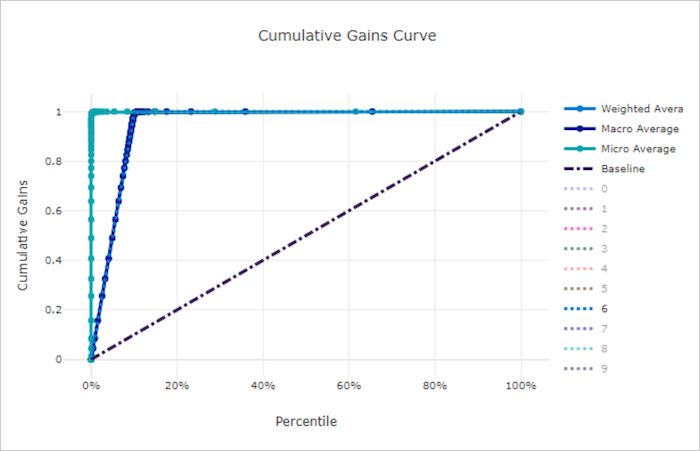### 不良模型的累积增益曲线Cumulative gains curve for a bad model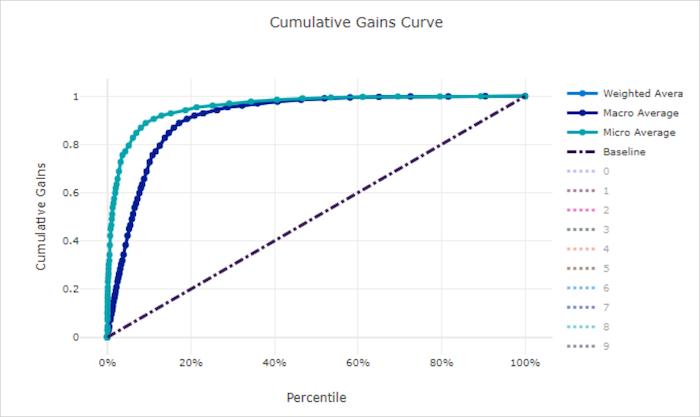## 提升曲线Lift curve

提升曲线显示某个模型的表现优于随机模型的次数。The lift curve shows how many times better a model performs compared to a random model. 提升定义为随机模型的累积增益与累积增益之比。Lift is defined as the ratio of cumulative gain to the cumulative gain of a random model.

这种相对表现考虑到类的数量越多，分类越困难。This relative performance takes into account the fact that classification gets harder as you increase the number of classes. （与具有两个类的数据集相比，随机模型对具有 10 个类的数据集中的样本进行预测时，错误率更高）(A random model incorrectly predicts a higher fraction of samples from a dataset with 10 classes compared to a dataset with two classes)

基线提升曲线是模型性能与随机模型性能一致的 `y = 1` 线。The baseline lift curve is the `y = 1` line where the model performance is consistent with that of a random model. 通常，良好模型的提升曲线在图表上会更高，离 x 轴更远，这表明当模型对其预测最有信心时，它的表现会比随机猜测好很多倍。In general, the lift curve for a good model will be higher on that chart and farther from the x-axis, showing that when the model is most confident in its predictions it performs many times better than random guessing.

提示

对于分类实验，为自动化 ML 模型生成的每个折线图都可以用于评估每个类的模型或所有类的平均值。For classification experiments, each of the line charts produced for automated ML models can be used to evaluate the model per-class or averaged over all classes. 通过单击图表右侧图例中的类标签，可以在这些不同的视图之间切换。You can switch between these different views by clicking on class labels in the legend to the right of the chart.

### 良好模型的提升曲线Lift curve for a good model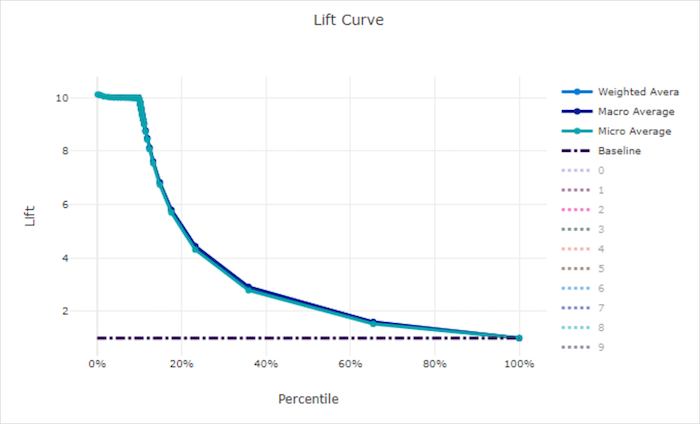### 不良模型的提升曲线Lift curve for a bad model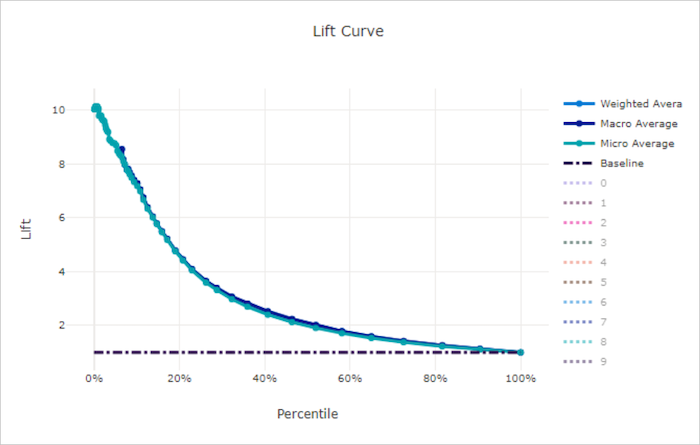## 校准曲线Calibration curve

校准曲线根据每个置信度水平的正样本比例绘制模型预测的置信度。The calibration curve plots a model's confidence in its predictions against the proportion of positive samples at each confidence level. 经过良好校准的模型将正确地对 100% 的预测进行分类，其中 100% 的预测将赋予 100% 的置信度，50% 的预测降赋予 50% 的置信度，20% 的预测将赋予 20% 的置信度，依此类推。A well-calibrated model will correctly classify 100% of the predictions to which it assigns 100% confidence, 50% of the predictions it assigns 50% confidence, 20% of the predictions it assigns a 20% confidence, and so on. 完全校准的模型将有一条校准曲线，沿着 `y = x` 线，模型完美地预测样本属于每个类别的概率。A perfectly calibrated model will have a calibration curve following the `y = x` line where the model perfectly predicts the probability that samples belong to each class.

置信度过高的模型在预测接近零和一的概率时会出现高估的情况，但很少出现无法确定每个样本的类的情况，并且校准曲线会类似于倒着的“S”。An over-confident model will over-predict probabilities close to zero and one, rarely being uncertain about the class of each sample and the calibration curve will look similar to backward "S". 置信度过低的模型将为其预测的类别平均分配较低的概率，相关的校准曲线将类似于“S”。An under-confident model will assign a lower probability on average to the class it predicts and the associated calibration curve will look similar to an "S". 校准曲线并不能说明一个模型是否能正确分类，而是说明它是否能正确向预测分配置信度。The calibration curve does not depict a model's ability to classify correctly, but instead its ability to correctly assign confidence to its predictions. 如果模型正确地分配了低置信度和高不确定性，则不良模型仍然可具有好的校准曲线。A bad model can still have a good calibration curve if the model correctly assigns low confidence and high uncertainty.

备注

校准曲线对样本数量非常敏感，因此小型验证集可能会产生难以解释的干扰结果。The calibration curve is sensitive to the number of samples, so a small validation set can produce noisy results that can be hard to interpret. 这并不一定意味着模型没有进行正确校准。This does not necessarily mean that the model is not well-calibrated.

### 良好模型的校准曲线Calibration curve for a good model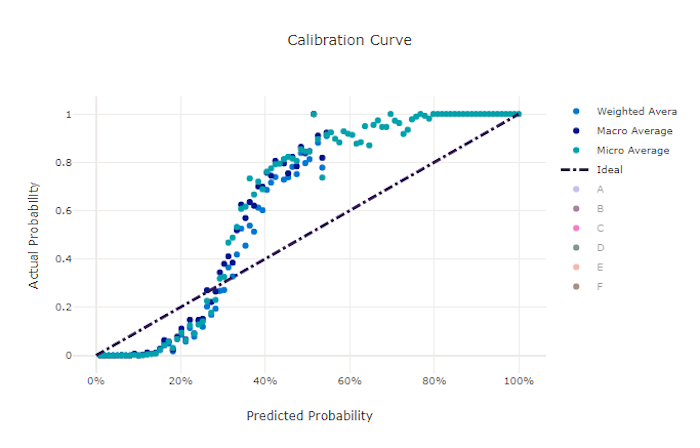### 不良模型的校准曲线Calibration curve for a bad model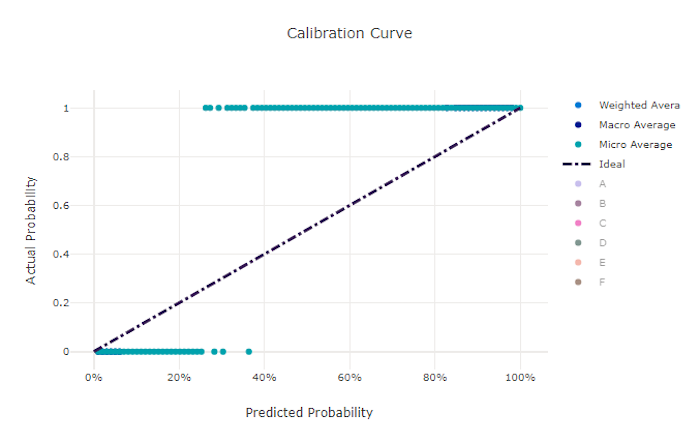## 回归/预测指标Regression/forecasting metrics

自动化 ML 为生成的每个模型计算相同的性能指标，不管它是回归试验还是预测试验。Automated ML calculates the same performance metrics for each model generated, regardless if it is a regression or forecasting experiment. 这些指标也经过规范化处理，以便在不同范围的数据上训练的模型之间进行比较。These metrics also undergo normalization to enable comparison between models trained on data with different ranges. 若要了解详细信息，请参阅指标规范化To learn more, see metric normalization.

下表总结了为回归和预测试验生成的模型性能指标。The following table summarizes the model performance metrics generated for regression and forecasting experiments. 与分类指标一样，这些指标也基于 scikit learn 实现。Like classification metrics, these metrics are also based on the scikit learn implementations. 相应的 scikit learn 文档在“计算”字段中链接。The appropriate scikit learn documentation is linked accordingly, in the Calculation field.

指标Metric 说明Description 计算Calculation
explained_varianceexplained_variance 解释的方差衡量模型对目标变量变化的解释程度。Explained variance measures the extent to which a model accounts for the variation in the target variable. 它是原始数据方差与误差方差之间的递减百分比。It is the percent decrease in variance of the original data to the variance of the errors. 当误差的平均值为 0 时，它等于确定系数（请参见下面的 r2_score）。When the mean of the errors is 0, it is equal to the coefficient of determination (see r2_score below).

目的： 越接近 1 越好Objective: Closer to 1 the better
范围： (-inf, 1]Range: (-inf, 1]
计算Calculation
mean_absolute_errormean_absolute_error 平均绝对误差是目标与预测之间的差的预期绝对值。Mean absolute error is the expected value of absolute value of difference between the target and the prediction.

目的： 越接近 0 越好Objective: Closer to 0 the better
范围： [0, inf)Range: [0, inf)

类型：Types:
`mean_absolute_error`
`normalized_mean_absolute_error`，mean_absolute_error 除以数据范围。`normalized_mean_absolute_error`, the mean_absolute_error divided by the range of the data.
计算Calculation
mean_absolute_percentage_errormean_absolute_percentage_error 平均绝对百分比误差 (MAPE) 是预测值和实际值之间平均差值的度量值。Mean absolute percentage error (MAPE) is a measure of the average difference between a predicted value and the actual value.

目的： 越接近 0 越好Objective: Closer to 0 the better
范围： [0, inf)Range: [0, inf)
median_absolute_errormedian_absolute_error 平均绝对误差是目标与预测之间的所有绝对差的中间值。Median absolute error is the median of all absolute differences between the target and the prediction. 此损失值可靠地反映离群值。This loss is robust to outliers.

目的： 越接近 0 越好Objective: Closer to 0 the better
范围： [0, inf)Range: [0, inf)

类型：Types:
`median_absolute_error`
`normalized_median_absolute_error`median_absolute_error 除以数据范围。`normalized_median_absolute_error`: the median_absolute_error divided by the range of the data.
计算Calculation
r2_scorer2_score R2（决定系数）衡量均方误差 (MSE) 相对于观察到的数据的总方差的按比例的降低程度。R2 (the coefficient of determination) measures the proportional reduction in mean squared error (MSE) relative to the total variance of the observed data.

目的： 越接近 1 越好Objective: Closer to 1 the better
范围： [-1, 1]Range: [-1, 1]

注意：R2 的范围通常为 (-inf, 1]。Note: R2 often has the range (-inf, 1]. MSE 可以大于观察到的方差，因此 R2 可以有任意大的负值，具体取决于数据和模型预测。The MSE can be larger than the observed variance, so R2 can have arbitrarily large negative values, depending on the data and the model predictions. 自动化 ML 剪辑报告的 R2 分数为 -1，因此 R2 的值为 -1 可能表示实际的 R2 分数小于 -1。Automated ML clips reported R2 scores at -1, so a value of -1 for R2 likely means that the true R2 score is less than -1. 在解释负 R2 分数时，请考虑其他指标值和数据的属性。Consider the other metrics values and the properties of the data when interpreting a negative R2 score.
计算Calculation
root_mean_squared_errorroot_mean_squared_error 均方根误差 (RMSE) 是目标与预测之间的预期平方差的平方根。Root mean squared error (RMSE) is the square root of the expected squared difference between the target and the prediction. 对于无偏差估算器，RMSE 等于标准偏差。For an unbiased estimator, RMSE is equal to the standard deviation.

目的： 越接近 0 越好Objective: Closer to 0 the better
范围： [0, inf)Range: [0, inf)

类型：Types:
`root_mean_squared_error`
`normalized_root_mean_squared_error`：root_mean_squared_error 除以数据范围。`normalized_root_mean_squared_error`: the root_mean_squared_error divided by the range of the data.
计算Calculation
root_mean_squared_log_errorroot_mean_squared_log_error 均方根对数误差是预期平方对数误差的平方根。Root mean squared log error is the square root of the expected squared logarithmic error.

目的： 越接近 0 越好Objective: Closer to 0 the better
范围： [0, inf)Range: [0, inf)

类型：Types:
`root_mean_squared_log_error`
`normalized_root_mean_squared_log_error`，root_mean_squared_log_error 除以数据范围。`normalized_root_mean_squared_log_error`: the root_mean_squared_log_error divided by the range of the data.
计算Calculation
spearman_correlationspearman_correlation 斯皮尔曼相关是两个数据集之间的关系单一性的非参数测量法。Spearman correlation is a nonparametric measure of the monotonicity of the relationship between two datasets. 与皮尔逊相关不同，斯皮尔曼相关不假设两个数据集呈正态分布。Unlike the Pearson correlation, the Spearman correlation does not assume that both datasets are normally distributed. 与其他相关系数一样，斯皮尔曼在 -1 和 +1 之间变化，0 表示不相关。Like other correlation coefficients, Spearman varies between -1 and 1 with 0 implying no correlation. -1 或 1 相关表示确切的单一关系。Correlations of -1 or 1 imply an exact monotonic relationship.

斯皮尔曼是一个秩相关指标，这意味着，如果预测值或实际值的变化不改变预测值或实际值的秩序，则不会改变斯皮尔曼结果。Spearman is a rank-order correlation metric meaning that changes to predicted or actual values will not change the Spearman result if they do not change the rank order of predicted or actual values.

目的： 越接近 1 越好Objective: Closer to 1 the better
范围： [-1, 1]Range: [-1, 1]
计算Calculation

### 指标规范化Metric normalization

自动化 ML 规范化回归和预测指标，使得在不同范围的数据上训练的模型之间能够进行比较。Automated ML normalizes regression and forecasting metrics which enables comparison between models trained on data with different ranges. 在较大范围的数据上训练的模型比在较小范围的数据上训练的模型具有更高的误差，除非该误差已规范化。A model trained on a data with a larger range has higher error than the same model trained on data with a smaller range, unless that error is normalized.

虽然没有规范化误差指标的方法，但是自动化 ML 采用的是将误差除以数据范围的通用方法：`normalized_error = error / (y_max - y_min)`While there is no standard method of normalizing error metrics, automated ML takes the common approach of dividing the error by the range of the data: `normalized_error = error / (y_max - y_min)`

在对时间序列数据的预测模型进行评估时，自动化 ML 会采取额外的步骤来确保按照时序 ID（粒度）进行规范化，因为每个时序可能具有不同的目标值分布。When evaluating a forecasting model on time series data, automated ML takes extra steps to ensure that normalization happens per time series ID (grain), because each time series likely has a different distribution of target values.

## 残差Residuals

残差图是为回归和预测试验生成的预测误差（残差）的直方图。The residuals chart is a histogram of the prediction errors (residuals) generated for regression and forecasting experiments. 所有样本的残差计算为 `y_predicted - y_true`，然后显示为直方图以显示模型偏差。Residuals are calculated as `y_predicted - y_true` for all samples and then displayed as a histogram to show model bias.

在本例中，请注意，两个模型的预测值都略低于实际值。In this example, note that both models are slightly biased to predict lower than the actual value. 对于实际目标分布扭曲的数据集来说，这并不罕见，但这表明模型性能较差。This is not uncommon for a dataset with a skewed distribution of actual targets, but indicates worse model performance. 良好的模型会有一个残差分布，在零处达到峰值，而在极值处残差很少。A good model will have a residuals distribution that peaks at zero with few residuals at the extremes. 更糟的模型会有一个分散的残差分布，在零附近有更少的样本。A worse model will have a spread out residuals distribution with fewer samples around zero.

### 良好模型的残差图Residuals chart for a good model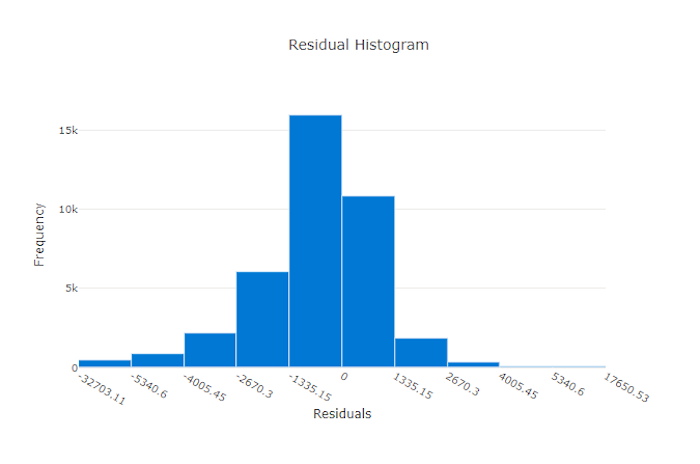### 不良模型的残差图Residuals chart for a bad model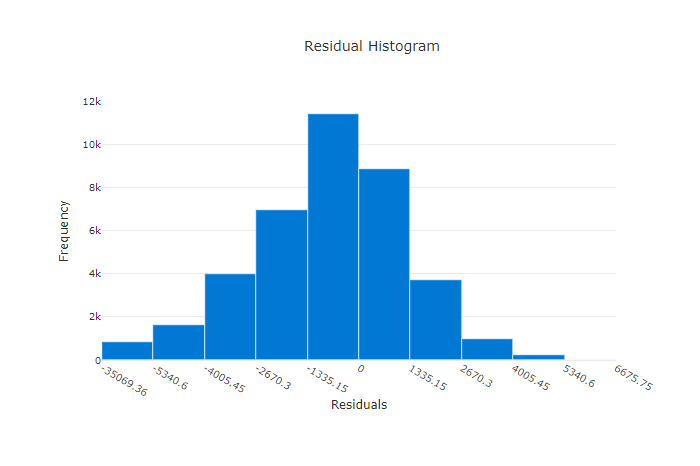## 预测值与实际值Predicted vs. true

对于回归和预测试验，预测与实际图表绘制了目标特征（实际值）与模型预测之间的关系。For regression and forecasting experiment the predicted vs. true chart plots the relationship between the target feature (true/actual values) and the model's predictions. 实际值沿 x 轴分格，每个分格的平均预测值用误差线绘制。The true values are binned along the x-axis and for each bin the mean predicted value is plotted with error bars. 这允许你查看模型是否偏向于预测某些值。This allows you to see if a model is biased toward predicting certain values. 该行显示平均预测，阴影区域表示围绕该平均值的预测方差。The line displays the average prediction and the shaded area indicates the variance of predictions around that mean.

通常，最常见的实际值将具有最准确的预测和最小的方差。Often, the most common true value will have the most accurate predictions with the lowest variance. 趋势线与理想 `y = x` 线之间的距离是一个很好的测量模型在异常值上的表现的方法。The distance of the trend line from the ideal `y = x` line where there are few true values is a good measure of model performance on outliers. 可以使用图表底部的直方图来推断实际的数据分布。You can use the histogram at the bottom of the chart to reason about the actual data distribution. 包括更多分布稀疏的数据样本可以提高模型对不可见数据的性能。Including more data samples where the distribution is sparse can improve model performance on unseen data.

在此示例中，请注意，更好的模型有一条更接近理想 `y = x` 线的预测与实际线。In this example, note that the better model has a predicted vs. true line that is closer to the ideal `y = x` line.

### 良好模型的预测与实际图表Predicted vs. true chart for a good model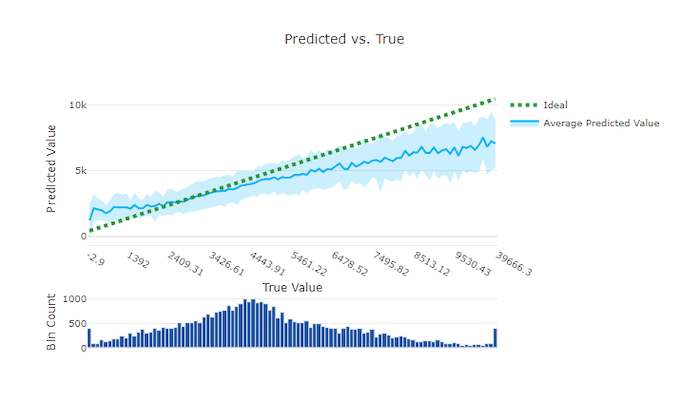### 不良模型的预测与实际图表Predicted vs. true chart for a bad model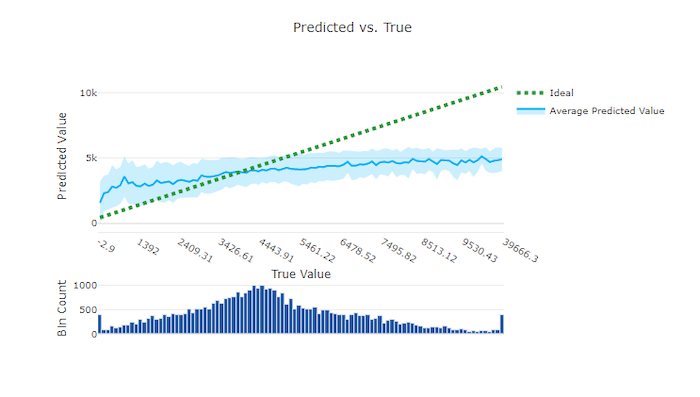## 模型解释和特征重要性Model explanations and feature importances

虽然模型评估指标和图表有助于衡量模型的总体质量，但在实践负责任的 AI 时，检查用于进行预测的模型的数据集特征是至关重要的。While model evaluation metrics and charts are good for measuring the general quality of a model, inspecting which dataset features a model used to make its predictions is essential when practicing responsible AI. 这就是自动化 ML 提供模型说明仪表板来测量和报告数据集特征的相对贡献的原因。That's why automated ML provides a model explanations dashboard to measure and report the relative contributions of dataset features. 请参阅如何在 Azure 机器学习工作室中查看说明仪表板

有关代码优先的体验，请参阅如何使用 Azure 机器学习 Python SDK 为自动 ML 试验设置模型说明For a code first experience, see how to set up model explanations for automated ML experiments with the Azure Machine Learning Python SDK.

备注

可解释性（最佳模型解释）不适用于将以下算法推荐为最佳模型或系综的自动化 ML 预测试验：Interpretability, best model explanation, is not available for automated ML forecasting experiments that recommend the following algorithms as the best model or ensemble:

• TCNForecasterTCNForecaster
• AutoArimaAutoArima
• ExponentialSmoothingExponentialSmoothing
• ProphetProphet
• 平均值Average
• NaiveNaive
• Seasonal AverageSeasonal Average
• Seasonal NaiveSeasonal Naive

## 后续步骤Next steps

• 请参阅自动化机器学习模型解释示例笔记本。
• 对于自动化 ML 特定问题，请联系 askautomatedml@microsoft.com。For automated ML specific questions, reach out to askautomatedml@microsoft.com.